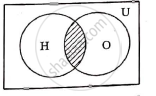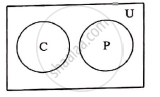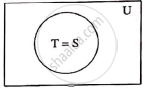# Express the Truth of Each of the Following Statements by Venn Diagram: (A) Some Hardworking Students Are Obedient. (B) No Circles Are Polygons. (C) All Teachers Are Scholars and Scholars Are Teachers. - Mathematics and Statistics

Sum

Express the truth of each of the following statements by Venn diagram:

(a) Some hardworking students are obedient.

(b) No circles are polygons.

(c) All teachers are scholars and scholars are teachers.

#### Solution

(a) Some hardworking students are obedient.

Let H: set of hardworking students

O: Set of obedient students

U: Set of all studentsfrom Venn diagram the truth value is H ∩ O

(b) No circles are polygons.

Let C: Set of all circles

P: Set of all polygons

U:set of all closed figuresFrom Venn diagram the truth value is

C ∩ P= Φ

(c) All teachers are scholars and scholars are teachers.

Let T: Set of all teachers

S: Set of all scholars

U: Set of all human beingsFrom Venn diagram the truth value is T=S

Concept: Venn Diagrams
Is there an error in this question or solution?
2014-2015 (March)

Share